# AutocorrelationAbove: A plot of a series of 100 random numbers concealing a sinefunction. Below: The sine function revealed in a correlogram produced by autocorrelation.

Autocorrelation, also known as serial correlation, is the cross-correlation of a signal with itself. Informally, it is the similarity between observations as a function of the time lag between them. It is a mathematical tool for finding repeating patterns, such as the presence of a periodic signal obscured by noise, or identifying the missing fundamental frequency in a signal implied by itsharmonic frequencies. It is often used in signal processing for analyzing functions or series of values, such as time domain signals.

## Definitions

Different fields of study define autocorrelation differently, and not all of these definitions are equivalent. In some fields, the term is used interchangeably withautocovariance.

### Statistics

In statistics, the autocorrelation of a random process describes the correlationbetween values of the process at different times, as a function of the two times or of the time lag. Let X be some repeatable process, and i be some point in time after the start of that process. (i may be an integer for a discrete-time process or a real number for a continuous-time process.) Then Xi is the value (or realization) produced by a given runof the process at time i. Suppose that the process is further known to have defined values for mean μi and variance σi2 for all times i. Then the definition of the autocorrelation between times s and t is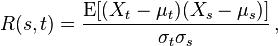$R(s,t) = \frac{\operatorname{E}[(X_t - \mu_t)(X_s - \mu_s)]}{\sigma_t\sigma_s}\, ,$

where “E” is the expected value operator. Note that this expression is not well-defined for all time series or processes, because the variance may be zero (for a constant process) or infinite. If the function R is well-defined, its value must lie in the range [−1, 1], with 1 indicating perfect correlation and −1 indicating perfect anti-correlation.

If Xt is a second-order stationary process then the mean μ and the variance σ2 are time-independent, and further the autocorrelation depends only on the lag between t and s: the correlation depends only on the time-distance between the pair of values but not on their position in time. This further implies that the autocorrelation can be expressed as a function of the time-lag, and that this would be an even function of the lag τ = s − t. This gives the more familiar form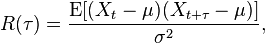$R(\tau) = \frac{\operatorname{E}[(X_t - \mu)(X_{t+\tau} - \mu)]}{\sigma^2}, \,$

and the fact that this is an even function can be stated as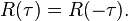$R(\tau) = R(-\tau).\,$

It is common practice in some disciplines, other than statistics and time series analysis, to drop the normalization by σ2 and use the term “autocorrelation” interchangeably with “autocovariance”. However, the normalization is important both because the interpretation of the autocorrelation as a correlation provides a scale-free measure of the strength of statistical dependence, and because the normalization has an effect on the statistical properties of the estimated autocorrelations.

### Signal processing

In signal processing, the above definition is often used without the normalization, that is, without subtracting the mean and dividing by the variance. When the autocorrelation function is normalized by mean and variance, it is sometimes referred to as the autocorrelation coefficient.

Given a signal$f(t)$, the continuous autocorrelation$R_{ff}(\tau)$ is most often defined as the continuous cross-correlation integral of$f(t)$ with itself, at lag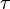$\tau$.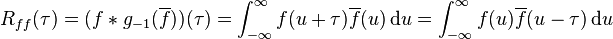$R_{ff}(\tau) = (f * g_{-1}(\overline{f}))(\tau) = \int_{-\infty}^\infty f(u+\tau)\overline{f}(u)\, {\rm d}u = \int_{-\infty}^\infty f(u)\overline{f}(u-\tau)\, {\rm d}u$

where$\overline{f}$ represents the complex conjugate,$g_{-1}$ is a function which manipulates the function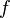$f$ and is defined as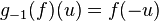$g_{-1}(f)(u)=f(-u)$ and$*$ represents convolution.

For a real function,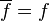$\overline{f} = f$.

Note that the parameter$u$ in the integral is a dummy variable and is only necessary to calculate the integral. It has no specific meaning.
The discrete autocorrelation$R$ at lag$l$ for a discrete signal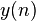$y(n)$ is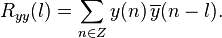$R_{yy}(l) = \sum_{n \in Z} y(n)\,\overline{y}(n-l).$

The above definitions work for signals that are square integrable, or square summable, that is, of finite energy. Signals that “last forever” are treated instead as random processes, in which case different definitions are needed, based on expected values. Forwide-sense-stationary random processes, the autocorrelations are defined as$R_{ff}(\tau) = \operatorname{E}\left[f(t)\overline{f}(t-\tau)\right]$$R_{yy}(l) = \operatorname{E}\left[y(n)\,\overline{y}(n-l)\right].$

For processes that are not stationary, these will also be functions of$t$, or$n$.

For processes that are also ergodic, the expectation can be replaced by the limit of a time average. The autocorrelation of an ergodic process is sometimes defined as or equated to$R_{ff}(\tau) = \lim_{T \rightarrow \infty} \frac{1}{T} \int_0^T f(t+\tau)\overline{f}(t)\, {\rm d}t$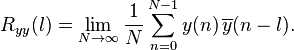$R_{yy}(l) = \lim_{N \rightarrow \infty} \frac{1}{N} \sum_{n=0}^{N-1}y(n)\,\overline{y}(n-l).$

These definitions have the advantage that they give sensible well-defined single-parameter results for periodic functions, even when those functions are not the output of stationary ergodic processes.

Alternatively, signals that last forever can be treated by a short-time autocorrelation function analysis, using finite time integrals. (Seeshort-time Fourier transform for a related process.)

Multi-dimensional autocorrelation is defined similarly. For example, in three dimensions the autocorrelation of a square-summablediscrete signal would be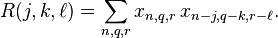$R(j,k,\ell) = \sum_{n,q,r} x_{n,q,r}\,x_{n-j,q-k,r-\ell}.$

When mean values are subtracted from signals before computing an autocorrelation function, the resulting function is usually called an auto-covariance function.

This site uses Akismet to reduce spam. Learn how your comment data is processed.